## Thursday, May 15, 2008

### Irodov Problem 1.160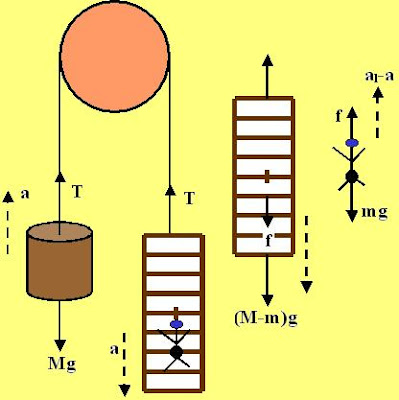This is a particularly interesting problem and so I decided to solve this in two methods, i) using force equations and ii) using conservation of momentum. Both these methods will ultimately give the same answer, but I thought solving using both these methods will show how everything fits snugly in the framework of physics.

Since the mass of the man in m and the counterweight is of mass M, this means that the mass of the ladder is M-m.

Solution Using Newtons Laws: To climb, the man must exert a force on the ladder using his muscles. The ladder then pushes him back with an equal and opposite reaction force. The force acting on the ladder due to the man's push makes the ladder move down while the reaction force from the ladder on the man makes him move up. As the ladder moves down, since the length of the string is conserved, the counterweight mass M moves up. This is essentially what happens.

Suppose that at some instant of time t the person exerts a force f(t) on the ladder (this can be some arbitrary function). Suppose that due to this force, the ladder is pushed down with an acceleration a(t) and the man climbs up with an acceleration al(t) upwards relative to the ladder. Let the tension in the string at some instant of time be T(t).

There are two forces acting on the counterweight mass M, i) the force of gravity Mg and ii) tension T(t). As the ladder moves down with an acceleration a(t), the counterweight will move upwards with an acceleration a(t). So we have,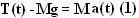There are three forces acting on the ladder, i) the force of gravity (M-m)g, ii) the tension T(t) and iii) the force f(t) exerted by the man on the ladder. The ladder moves down with an acceleration a(t). So we have,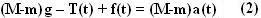There are two forces acting on the man, i) the force of gravity mg and ii) the reaction force f(t) offered by the ladder. The man moves down along with the ladder with an acceleration a(t) and relative to the ladder with an acceleration al(t). So the net acceleration of the man with respect to a stationary observer is al(t)-a(t) upwards. So we have,Simply adding (1) and (2) we have,From (3) and (4) we can writeFrom this we have,What (6) tells us is that if the man moves up relative to the ladder by a value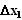then the ladder will move down a distance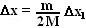. This will cause the counterweight mass M to move up byunits. Since the man movesrelative to the ladder, but the ladder itself movesunits down, his net movement relative to a stationary reference frame will be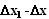upwards.

The net movement of the CG of the system will thus be,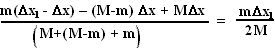Since in the question the distance moved by the person relative to the ladder is l' the net displacement of the CG of the system will be ml'/2M.

Solution Using Conservation of Momentum:In the figure beside, System A is the original system in the problem. Let us do a simple transformation. Remove the pulley and stretch the string into a straight line. We shall call this System B. Now, System B is acted upon by two external forces i) Mg pulling the counterweight to the left and ii) Mg pulling the (Man + ladder) to the right. So the net force on the entire system is 0. So we can actually reduce System B into System C where we simply remove the force of gravity. Behavior of System C will be identical to that of System A.

Now since there are no external forces on entire System C its momentum must be conserved. Since the entire System C was static before the man started to move, the center of mass (CG in the figure) of System C will never move. Suppose that when the man moved a distance l' to the left relative to the ladder, the counterweight M and the ladder moved a distance y to the right. Since the man also moved y units to the right with the ladder his net movement of the man will be (l'-y). The net movement of the CG of System C is then given by,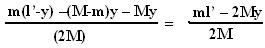.

But we know that the CG of System C will not displace and so we have,This is exactly same as what equation (6) says - if the man moves a distance l' relative to the ladder, the ladder and the counterweight will move a distance. Since System A and C will behave exactly the same way, even in System C if the man climbs up l' units, the ladder will moveunits down and the counterweight M will moveup.

So the net movement of the CG of System A will be,This is exactly what the solution predicted when we solved the question using newton's laws!!